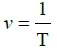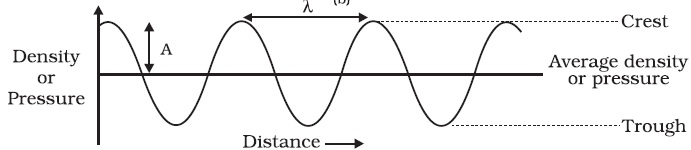Study Materials: CBSE Notes

Our CBSE Notes for Notes 12. Sound - Sound Wave | Class 9 Science - Toppers Study is the best material for English Medium students cbse board and other state boards students.

Notes ⇒ Class 9th ⇒ Science ⇒ 12. Sound

# Notes 12. Sound - Sound Wave | Class 9 Science - Toppers Study

Topper Study classes prepares CBSE Notes on practical base problems and comes out with the best result that helps the students and teachers as well as tutors and so many ecademic coaching classes that they need in practical life. Our CBSE Notes for Notes 12. Sound - Sound Wave | Class 9 Science - Toppers Study is the best material for English Medium students cbse board and other state boards students.

## Notes 12. Sound - Sound Wave | Class 9 Science - Toppers Study

CBSE board students who preparing for class 9 ncert solutions maths and Science solved exercise chapter 12. Sound available and this helps in upcoming exams 2023-2024.

### You can Find Science solution Class 9 Chapter 12. Sound

• All Chapter review quick revision notes for chapter 12. Sound Class 9
• NCERT Solutions And Textual questions Answers Class 9 Science
• Extra NCERT Book questions Answers Class 9 Science
• Importatnt key points with additional Assignment and questions bank solved.

Chapter 12 Science class 9

### Sound Wave class 9 Science Chapter 12. Sound

• Notes 12. Sound - Sound Wave | Class 9 Science - Toppers Study
• Class 9 Ncert Solutions
• Solution Chapter 12. Sound Class 9
• Solutions Class 9
• Chapter 12. Sound Sound Wave Class 9

## Notes 12. Sound - Sound Wave | Class 9 Science - Toppers Study

Sound Wave : Sound is known as the wave and wave is disturbance which is produced by a vibrating object. These wave are longitudinal wave which create by compression and rarefaction.

Sound wave is mechanical wave: Sound waves need medium to propagate from one place to another, in otherhand it need body for transmission, this is the reason that sound waves are called mechanical waves.

Common medium for the propagation of sound : Air is common medium.

Sound waves are longitudinal waves:

Sound wavves are longitudinal waves because the displacement of particles of medium occure parallel to the direction of propagation in these waves. The particles do not move from one place to another but they oscillate in to and fro motion ramain about mean position as the waves travel. Just like this sound waves propagate, therefore sound waves are longitudinal waves.

Features of sound waves:

There are following features of sound waves:

(i) Frequency

(ii) Amplitude

(iii) Velocity

Wavelength : The distance between two consecutive compressions (C) or two consecutive rarefactions (R) is called the wavelength.

Or the distance covered by a wave to complete an oscillation is called wavelength.

Hence wavelength is kind of length, therefore the S.I unit of wavelength is metre (m).

Wavelength is represented by Greek letter λ (lambda).

Frequency: The number of oscillation completed in one second is called frequency.

S.I unit of frequncy is Hertz(Hz).

Time Period : The time taken to complete one oscillation is called it's time period.

• SI unit of time period is second (s). Time period is denoted by T.
• The frequency of a wave is the reciprocal of the time period.Amplitude : The maximum displacement of the particles of medium from their original undisturbed position (equilibrium position) during the propagation of wave is called amplitude.

Amplitude is denoted by A and its SI unit is metre (m).

Representing graphically the density and pressure variations.Sound have characteristics like pitch, loudness and timbre.

Crest: A pick of sound wave is called crest.

Trough: A valley of the sound wave is called trough.

Timbre : The quality or timber of sound is that characteristic which enables us to distinguish one sound from another having the same pitch and loudness.

Tone : A sound of single frequency is called a tone.

Note : The sound which is produced due to a mixture of several frequencies is called a note and is pleasant to listen.

Noise: The sound which is unpleasant to ear is called noise.

The speed of sound : The speed of sound is defined as the distance which a point on a wave, such as a compression or a rarefaction, travels per unit time.

Speed (v) = distance/timeλ is wavelength

T is time period

or v = λ ν
That is, speed = wavelength × frequency.

• The speed of sound remains almost the same for all frequencies in a given medium under the same physical conditions.

Example 1. A sound wave has a frequency of 2 kHz and wave length
35 cm. Find the speed of sound.

Solution:

Given,
Frequency, ν = 2 kHz = 2000 Hz
Wavelength, λ = 35 cm = 0.35 m

Speed (v) = wavelength × frequency

= λ ν

= 0.35 × 2000

= 700 m/s

Therefore speed is 700 m/s

Example 2. Calculate the wavelength of a sound wave whose frequency is 220 Hz and speed is 440 m/s in a given medium.

Solution:

Frequency = 220 Hz

Speed = 440 m/s

Wavelength = speed/frequency

= 440/220

= 2 m

Therefore, wavelength is 2 m

Intensity of sound : The amount of sound energy passing each second through unit area is called the intensity of sound.

Loudness of sound : Loudness is a measure of the response of the
ear to the sound.

Factors on which the loudness of sound depends:

(i) Loudness of sound depends on apletudes of vibration.

The different between transverse wave and longitudinal wave:
Transverse wave:
1. Particles of the medium moves in a direction parallel to the direction of propagation of the disturbance.
2. It forms compression and rarefaction.
3. Example – light wave.
Longitudinal wave:
1. Particles do not oscillate along the line of wave propagation but oscillate up and down about their mean position as the wave travels
2. It forms crest and trough.
3. Example – sound wave.

Important Study materials for classes 06, 07, 08,09,10, 11 and 12. Like CBSE Notes, Notes for Science, Notes for maths, Notes for Social Science, Notes for Accountancy, Notes for Economics, Notes for political Science, Noes for History, Notes For Bussiness Study, Physical Educations, Sample Papers, Test Papers, Mock Test Papers, Support Materials and Books.Mathematics Class - 11th

NCERT Maths book for CBSE Students.

books

## Study Materials List:

##### Notes ⇒ Class 9th ⇒ Science
1. Matter in Our Surroundings
2. Is Matter around us Pure
3. Atoms and Molecules
4. Structure of The Atom
5. The Fundamental Unit of Life
6. Tissues
7. Diversity in Living Organisms
8. Motion
9. Force and Laws of Motion
10. Gravitation
11. Work and Energy
12. Sound
13. Why Do We Fall ill
14. Natural Resources
15. Improvement in Food Resources

New Books